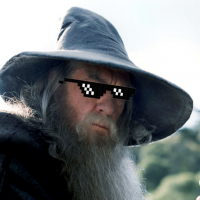# Incorrect calculation when computing the value using case statement

Hi,

The below beast mode in DOMO gives correct results when only 'metric A' is used as a filter in domo.

When 'metric A' and 'metric B' is selected as a filter, the computed result goes a bit off.

I have attached screenshots of the result.

Note: The numbers changes for 5th Dec'22. Correct value is 72224.29 when used only 'metric A', in case of 2 metrics in filter the result changes to 72213.86

```(case
when `metrics`=`metric A`
then
(case
when ((`values` - lag(`values`,1) OVER (ORDER BY `date_id`))=0 and `date_id`>'2022-10-01' )
then (((lag(`values`,1) OVER (ORDER BY `date_id`)) - (lag(`values`,7) OVER (ORDER BY `date_id`)))/7 + `values`)
else `values`
END
)
end)
```

•Contributor